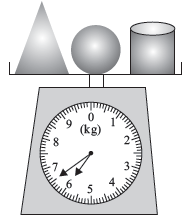# User ForumSubject :IMO    Class : Class 5

The total weight of a ball, a cylinder and a cone is shown in the given figure. The ball is 2 kg heavier than the cone. The cylinder is 1.5 kg heavier than the cone.What is the weight of the cone?A1 kg
B2 kg
C3 kg
D4 kg

Class : Class 5

## Ans 2:

Class : Class 6
Ans is A. Think the weight of the cone is x. x+[(x+2 kg)+(x+1.5 kg)=x+(2x+3.5 kg)=3x+3.5 kg=6.5 kg. So x will be (6.5 kg-3.5 kg)/3=3 kg/3=1 kg.